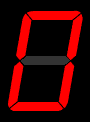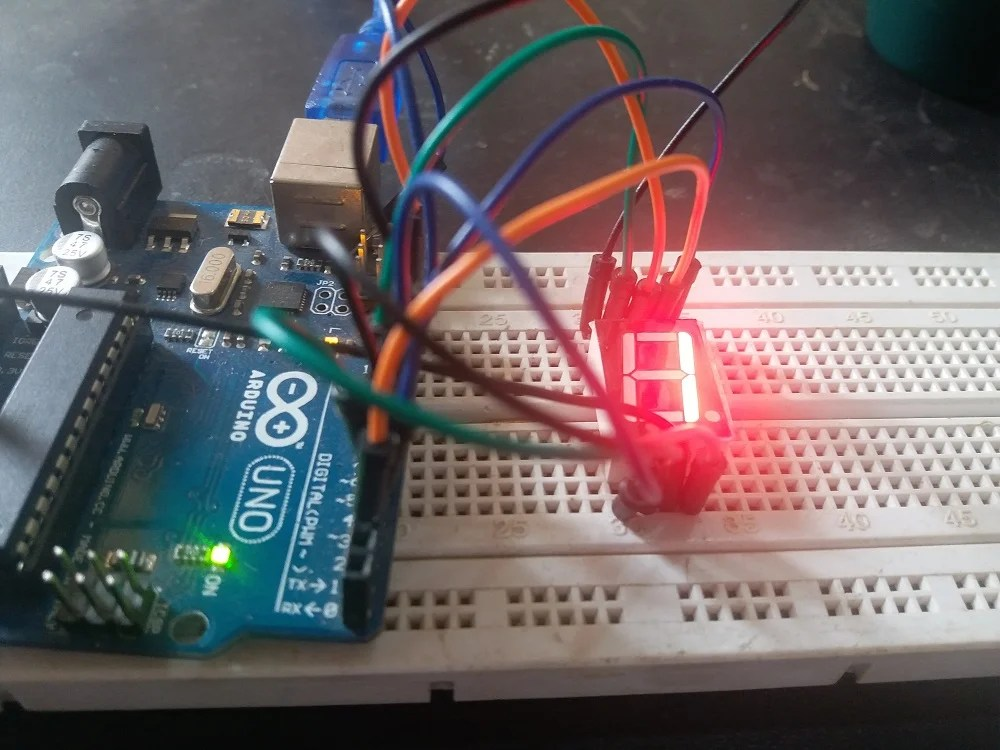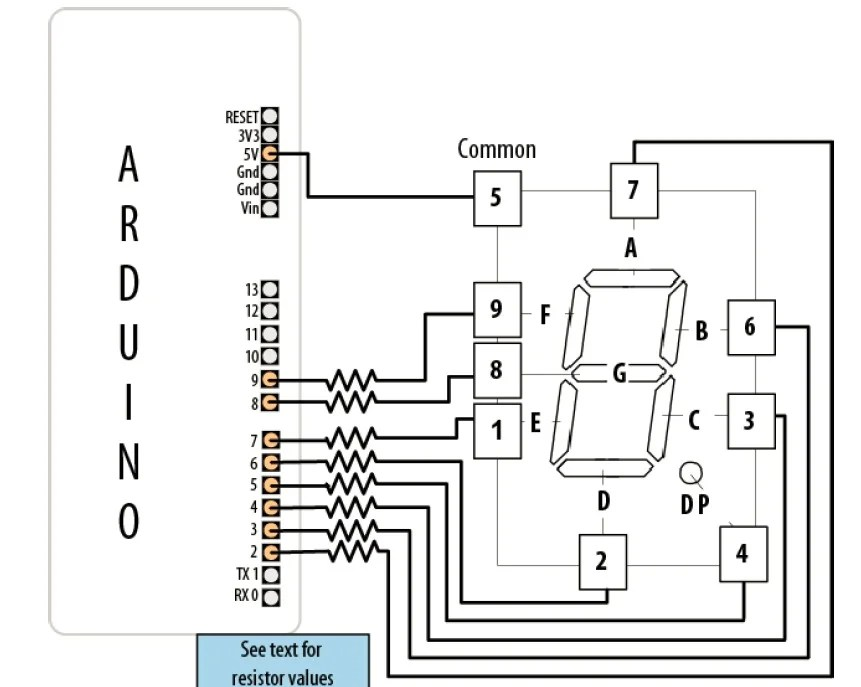# Seven segment display interfacing with Arduino: Multiple

In this tutorial, you will learn how to interface seven segment display with Arduino? How to interface multiple seven segment displays with Arduino? Seven segment displays are used to display numeric values of many types of sensors. For example, if you are designing a ac voltmeter or ac current meter using Arduino , you can use seven segment display instead of 16X2 liquid crystal display to display voltage and current values. So seven segment display has many applications in embedded systems  and Arduino based projects.

First of all if you need to know how to interface multiple seven segment displays with Arduino, you should know how to use digital pins of Arduino as a digital output. In other words, you should know how to use input and output ports of Arduino.### What is seven segment display?

So first of all lets see what is seven segment display? What is internal construction of a single seven segment display. It is mostly used in electronics circuits, electronics calculators, digital displays and many other electronics devices. The 7 segment display consists of 7 light emitting diodes. These light emitting diodes are arranged in such a way that by turning on and turning off specific pattern of LEDs we can display a number on unique number on seven segment display as shown in figure above. There is a also a extra eighth LED on seven segment display which is used to display dot. There are types of seven segment displays are available in market.

### Single Seven segment display interfacing with ArduinoFigure below shows, how to make connections with Arduino and seven segment display. Common anode types seven segment display is used in this tutorial and as you can see we have connected 5 volt with with common pin of seven segment display. Digital output pins of Arduino from pin number 2 to pin number 9 are connected with seven segment display.### Code of seven segment display interfacing with Arduino

```// bits representing segments A through G (and decimal point) for numerals 0-9
const byte numeral = {
//ABCDEFG /dp
B11111100, // 0
B01100000, // 1
B11011010, // 2
B11110010, // 3
B01100110, // 4
B10110110, // 5
B00111110, // 6
B11100000, // 7
B11111110, // 8
B11100110, // 9
};
// pins for decimal point and each segment
// dp,G,F,E,D,C,B,A
const int segmentPins = { 5,9,8,7,6,4,3,2};
void setup()
{
for(int i=0; i < 8; i++)
{
pinMode(segmentPins[i], OUTPUT); // set segment and DP pins to output
}
}
void loop()
{
for(int i=0; i <= 10; i++)
{
showDigit(i);
delay(1000);
}
// the last value if i is 10 and this will turn the display off
delay(2000); // pause two seconds with the display off
}
// Displays a number from 0 through 9 on a 7-segment display
// any value not within the range of 0-9 turns the display off
void showDigit( int number)
{
boolean isBitSet;
for(int segment = 1; segment < 8; segment++)
{
if( number < 0 || number > 9){
isBitSet = 0; // turn off all segments
}
else{
// isBitSet will be true if given bit is 1
isBitSet = bitRead(numeral[number], segment);
}
isBitSet = ! isBitSet; // remove this line if common cathode display
digitalWrite( segmentPins[segment], isBitSet);
}
}```

so this code shows the numeric values on seven segment display.